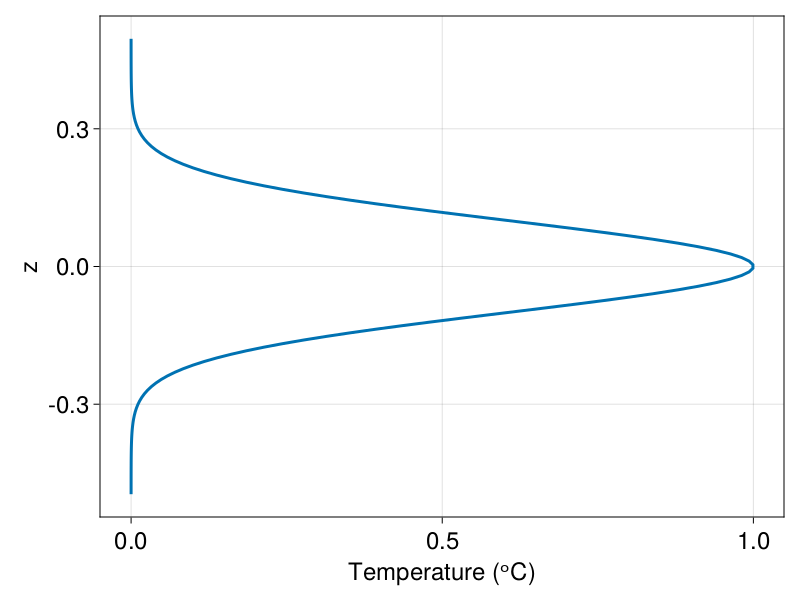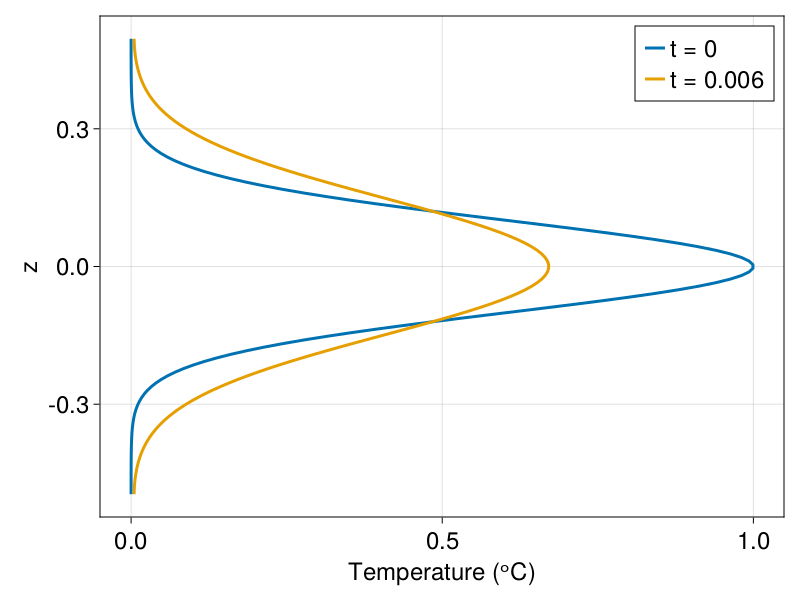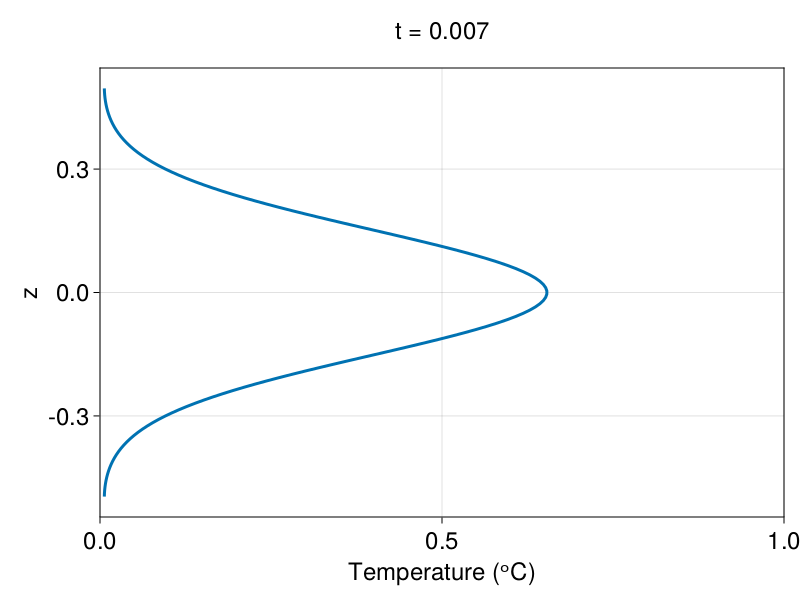# Simple diffusion example

This is Oceananigans.jl's simplest example: the diffusion of a one-dimensional Gaussian. This example demonstrates

• How to load Oceananigans.jl.
• How to instantiate an Oceananigans.jl model.
• How to create simple Oceananigans.jl output.
• How to set an initial condition with a function.
• How to time-step a model forward.
• How to look at results.

## Install dependencies

First let's make sure we have all required packages installed.

using Pkg
pkg"add Oceananigans, CairoMakie"

## Using Oceananigans.jl

Write

using Oceananigans

to load Oceananigans functions and objects into our script.

## Instantiating and configuring a model

A core Oceananigans type is NonhydrostaticModel. We build a NonhydrostaticModel by passing it a grid, plus information about the equations we would like to solve.

Below, we build a rectilinear grid with 128 regularly-spaced grid points in the z-direction, where z spans from z = -0.5 to z = 0.5,

grid = RectilinearGrid(size=128, z=(-0.5, 0.5), topology=(Flat, Flat, Bounded))
1×1×128 RectilinearGrid{Float64, Flat, Flat, Bounded} on CPU with 0×0×3 halo
├── Flat x
├── Flat y
└── Bounded  z ∈ [-0.5, 0.5]      regularly spaced with Δz=0.0078125

The default topology is (Periodic, Periodic, Bounded). In this example, we're trying to solve a one-dimensional problem, so we assign Flat to the x and y topologies. We excise halos and avoid interpolation or differencing in Flat directions, saving computation and memory.

We next specify a model with an ScalarDiffusivity, which models either molecular or turbulent diffusion,

closure = ScalarDiffusivity(κ=1)
ScalarDiffusivity{ExplicitTimeDiscretization}(ν=0.0, κ=1.0)

We finally pass these two ingredients to NonhydrostaticModel,

model = NonhydrostaticModel(; grid, closure, tracers=:T)
NonhydrostaticModel{CPU, RectilinearGrid}(time = 0 seconds, iteration = 0)
├── grid: 1×1×128 RectilinearGrid{Float64, Flat, Flat, Bounded} on CPU with 0×0×3 halo
├── tracers: T
├── closure: ScalarDiffusivity{ExplicitTimeDiscretization}(ν=0.0, κ=(T=1.0,))
├── buoyancy: Nothing
└── coriolis: Nothing

By default, NonhydrostaticModel has no-flux (insulating and stress-free) boundary conditions on all fields.

Next, we set! an initial condition on the temperature field, model.tracers.T. Our objective is to observe the diffusion of a Gaussian.

width = 0.1
initial_temperature(x, y, z) = exp(-z^2 / (2width^2))
set!(model, T=initial_temperature)

## Visualizing model data

Calling set! above changes the data contained in model.tracers.T, which was initialized as 0's when the model was created. To see the new data in model.tracers.T, we plot it:

using CairoMakie
set_theme!(Theme(fontsize = 24, linewidth=3))

fig = Figure()
axis = (xlabel = "Temperature (ᵒC)", ylabel = "z")
label = "t = 0"

z = znodes(model.tracers.T)
T = interior(model.tracers.T, 1, 1, :)

lines(T, z; label, axis)The function interior above extracts a view of model.tracers.T over the physical points (excluding halos) at (1, 1, :).

## Running a Simulation

Next we set-up a Simulation that time-steps the model forward and manages output.

# Time-scale for diffusion across a grid cell
diffusion_time_scale = model.grid.Δzᵃᵃᶜ^2 / model.closure.κ.T

simulation = Simulation(model, Δt = 0.1 * diffusion_time_scale, stop_iteration = 1000)
Simulation of NonhydrostaticModel{CPU, RectilinearGrid}(time = 0 seconds, iteration = 0)
├── Next time step: 6.104 μs
├── Elapsed wall time: 0 seconds
├── Wall time per iteration: NaN years
├── Stop time: Inf years
├── Stop iteration : 1000.0
├── Wall time limit: Inf
├── Callbacks: OrderedDict with 4 entries:
│   ├── stop_time_exceeded => Callback of stop_time_exceeded on IterationInterval(1)
│   ├── stop_iteration_exceeded => Callback of stop_iteration_exceeded on IterationInterval(1)
│   ├── wall_time_limit_exceeded => Callback of wall_time_limit_exceeded on IterationInterval(1)
│   └── nan_checker => Callback of NaNChecker for u on IterationInterval(100)
├── Output writers: OrderedDict with no entries
└── Diagnostics: OrderedDict with no entries

simulation will run for 1000 iterations with a time-step that resolves the time-scale at which our temperature field diffuses. All that's left is to

run!(simulation)
[ Info: Initializing simulation...
[ Info:     ... simulation initialization complete (195.282 ms)
[ Info: Executing initial time step...
[ Info:     ... initial time step complete (25.395 seconds).
[ Info: Simulation is stopping. Model iteration 1000 has hit or exceeded simulation stop iteration 1000.

## Visualizing the results

Let's look at how model.tracers.T changed during the simulation.

using Printf

label = @sprintf("t = %.3f", model.clock.time)
lines!(interior(model.tracers.T, 1, 1, :), z; label)
axislegend()Very interesting! Next, we run the simulation a bit longer and make an animation. For this, we use the JLD2OutputWriter to write data to disk as the simulation progresses.

simulation.output_writers[:temperature] =
JLD2OutputWriter(model, model.tracers,
filename = "one_dimensional_diffusion.jld2",
schedule=IterationInterval(100),
overwrite_existing = true)
JLD2OutputWriter scheduled on IterationInterval(100):
├── filepath: ./one_dimensional_diffusion.jld2
├── 1 outputs: T
├── array type: Array{Float32}
├── including: [:grid, :coriolis, :buoyancy, :closure]
└── max filesize: Inf YiB

We run the simulation for 10,000 more iterations,

simulation.stop_iteration += 10000
run!(simulation)
[ Info: Initializing simulation...
[ Info:     ... simulation initialization complete (48.728 ms)
[ Info: Executing initial time step...
[ Info:     ... initial time step complete (3.285 ms).
[ Info: Simulation is stopping. Model iteration 11000 has hit or exceeded simulation stop iteration 11000.

Finally, we animate the results by opening the JLD2 file, extract the iterations we ended up saving at, and plot the evolution of the temperature profile in a loop over the iterations.

T_timeseries = FieldTimeSeries("one_dimensional_diffusion.jld2", "T")
times = T_timeseries.times

fig = Figure()
ax = Axis(fig[2, 1]; xlabel = "Temperature (ᵒC)", ylabel = "z")
xlims!(ax, 0, 1)

n = Observable(1)

T = @lift interior(T_timeseries[$n], 1, 1, :) lines!(T, z) label = @lift "t = " * string(round(times[$n], digits=3))
Label(fig[1, 1], label, tellwidth=false)Finally, we record a movie.

frames = 1:length(times)

@info "Making an animation..."

record(fig, "one_dimensional_diffusion.mp4", frames, framerate=24) do i
msg = string("Plotting frame ", i, " of ", frames[end])
print(msg * " \r")
n[] = i
end
[ Info: Making an animation...
Plotting frame 1 of 100
Plotting frame 2 of 100
Plotting frame 3 of 100
Plotting frame 4 of 100
Plotting frame 5 of 100
Plotting frame 6 of 100
Plotting frame 7 of 100
Plotting frame 8 of 100
Plotting frame 9 of 100
Plotting frame 10 of 100
Plotting frame 11 of 100
Plotting frame 12 of 100
Plotting frame 13 of 100
Plotting frame 14 of 100
Plotting frame 15 of 100
Plotting frame 16 of 100
Plotting frame 17 of 100
Plotting frame 18 of 100
Plotting frame 19 of 100
Plotting frame 20 of 100
Plotting frame 21 of 100
Plotting frame 22 of 100
Plotting frame 23 of 100
Plotting frame 24 of 100
Plotting frame 25 of 100
Plotting frame 26 of 100
Plotting frame 27 of 100
Plotting frame 28 of 100
Plotting frame 29 of 100
Plotting frame 30 of 100
Plotting frame 31 of 100
Plotting frame 32 of 100
Plotting frame 33 of 100
Plotting frame 34 of 100
Plotting frame 35 of 100
Plotting frame 36 of 100
Plotting frame 37 of 100
Plotting frame 38 of 100
Plotting frame 39 of 100
Plotting frame 40 of 100
Plotting frame 41 of 100
Plotting frame 42 of 100
Plotting frame 43 of 100
Plotting frame 44 of 100
Plotting frame 45 of 100
Plotting frame 46 of 100
Plotting frame 47 of 100
Plotting frame 48 of 100
Plotting frame 49 of 100
Plotting frame 50 of 100
Plotting frame 51 of 100
Plotting frame 52 of 100
Plotting frame 53 of 100
Plotting frame 54 of 100
Plotting frame 55 of 100
Plotting frame 56 of 100
Plotting frame 57 of 100
Plotting frame 58 of 100
Plotting frame 59 of 100
Plotting frame 60 of 100
Plotting frame 61 of 100
Plotting frame 62 of 100
Plotting frame 63 of 100
Plotting frame 64 of 100
Plotting frame 65 of 100
Plotting frame 66 of 100
Plotting frame 67 of 100
Plotting frame 68 of 100
Plotting frame 69 of 100
Plotting frame 70 of 100
Plotting frame 71 of 100
Plotting frame 72 of 100
Plotting frame 73 of 100
Plotting frame 74 of 100
Plotting frame 75 of 100
Plotting frame 76 of 100
Plotting frame 77 of 100
Plotting frame 78 of 100
Plotting frame 79 of 100
Plotting frame 80 of 100
Plotting frame 81 of 100
Plotting frame 82 of 100
Plotting frame 83 of 100
Plotting frame 84 of 100
Plotting frame 85 of 100
Plotting frame 86 of 100
Plotting frame 87 of 100
Plotting frame 88 of 100
Plotting frame 89 of 100
Plotting frame 90 of 100
Plotting frame 91 of 100
Plotting frame 92 of 100
Plotting frame 93 of 100
Plotting frame 94 of 100
Plotting frame 95 of 100
Plotting frame 96 of 100
Plotting frame 97 of 100
Plotting frame 98 of 100
Plotting frame 99 of 100
Plotting frame 100 of 100